CAT  >  Important Formulas: Logarithms

# Important Formulas: Logarithms Notes | Study Quantitative Aptitude (Quant) - CAT

## Document Description: Important Formulas: Logarithms for CAT 2022 is part of Quantitative Aptitude (Quant) preparation. The notes and questions for Important Formulas: Logarithms have been prepared according to the CAT exam syllabus. Information about Important Formulas: Logarithms covers topics like Logarithm, Properties of Logarithms and Important Formulas: Logarithms Example, for CAT 2022 Exam. Find important definitions, questions, notes, meanings, examples, exercises and tests below for Important Formulas: Logarithms.

Introduction of Important Formulas: Logarithms in English is available as part of our Quantitative Aptitude (Quant) for CAT & Important Formulas: Logarithms in Hindi for Quantitative Aptitude (Quant) course. Download more important topics related with notes, lectures and mock test series for CAT Exam by signing up for free. CAT: Important Formulas: Logarithms Notes | Study Quantitative Aptitude (Quant) - CAT
 1 Crore+ students have signed up on EduRev. Have you?

Logarithm

• If a is a positive real number, other than 1 and am = x, then we write: m = loga x and we say that the value of log x to the base a is m.
• Examples: (i) 103 1000 ⇒ log10 1000 = 3.
(ii) 34 = 81 ⇒ log3 81 = 4.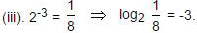(iv) (. 1)2 = 01 ⇒ log(.1) .01 = 2.

Properties of Logarithms

1. log a (xy) = loga x + loga y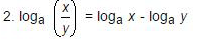3. logx x = 1

4. loga 1 = 0

5. loga (xn) = n(loga x)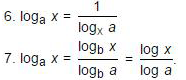Common Logarithms

• Logarithms to the base 10 are known as common logarithms.
• The logarithm of a number contains two parts, namely 'characteristic' and 'mantissa'.

1. Characteristic

The internal part of the logarithm of a number is called its characteristic.
Case I: When the number is greater than 1.

• In this case, the characteristic is one less than the number of digits in the left of the decimal point in the given number.

Case II: When the number is less than 1.

• In this case, the characteristic is one more than the number of zeros between the decimal point and the first significant digit of the number and it is negative.
• Instead of -1, -2 etc. we write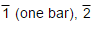(two bar), etc.
Examples: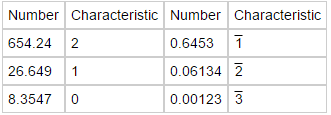2. Mantissa:

The decimal part of the logarithm of a number is known is its mantissa. For mantissa, we look through log table.

Question 1: If log2X + log4X = log0.25√6 and x > 0, then x is:

A. 6-1/6

B. 61/6

C. 3-1/3

D. 61/3

Correct Answer is Option (A).

• log2x + log4x = log0.25√6
We can rewrite the equation as: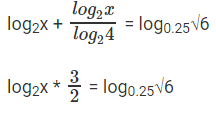log2x * 3 = 2log0.25√6
log2x3 = -log46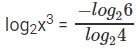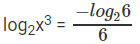2log2x3 = -log26
• 2log2x3 + log26 = 0
log26X6 = 0
• 6x6 = 1
x6 = 1/6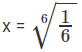• The question is "If log2X + log4X = log0.25 √6 and x > 0, then x is"
• Hence, the answer is "6-1/6".

Question 2: log9 (3log2 (1 + log3 (1 + 2log2x))) = 1/2. Find x.

A. 4

B. 1/2

C. 1

D. 2

Correct Answer is Option (D).

log9 (3log2 (1 + log3 (1 + 2log2x)) = 1/2

3log2(1 + log3(1 + 2log2x)) = 91/2 = 3
log2(1 + log3(1 + 2log2x) = 1
1 + log3(1 + 2log2x) = 2
log3(1 + 2log2x) = 1
1 + 2log2x = 3
2log2x = 2
log2x = 1
x = 2

The question is "Find x."

##### Hence, the answer is "2".

Question 3: If 22x+4 – 17 × 2x+1 = –4, then which of the following is true?

A. x is a positive value

B. x is a negative value

C. x can be either a positive value or a negative value

D. None of these

Correct Answer is Option (C).

2x+4 – 17 * 2x+1 = – 4
=> 2x+1 = y
22x+2 = y2
22(22x+2) – 17 * 2x+1 = –4
4y2 – 17y + 4 = 0
4y2 – 16y – y + y = 0
4y (y – 4) – 1 (y – 4) = 0

y = 1/4 or 4

2x+1 = 1/4 or 4

⇒ x + 1 = 2 or – 2
x = 1 or – 3

The question is "which of the following is true?"

##### Hence, the answer is "x can be either a positive value or a negative value".

Question 4: If log1227 = a, log916 = b, find log8108

A.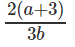B.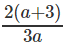C.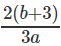D.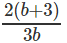Correct Answer is Option (D).

log8108 = log8(4 * 27)
log8108 = log84 + log827
log84 = 2/3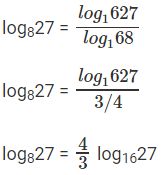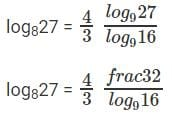log827 = 2 * log16
log916 = b
log169 = 1/b

log827 = 2/b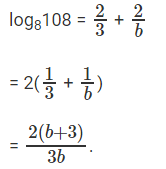The question is "find log8108."

##### Hence, the answer is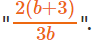Question 5: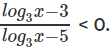If a, b are integers such that x = a, and x = b satisfy this inequation, find the maximum possible value of a – b.

A. 214

B. 216

C. 200

D. 203

Correct Answer is Option (A).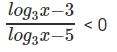log3x = y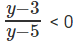y ∈ (3, 5)
3 < log3x < 5
27 < x < 243
Therefore max ( a – b) will be when a = 242 and b = 28. Therefore, max(a – b) = 214.

The question is "find the maximum possible value of a – b."

##### Hence, the answer is "214".

Question 6: log5x = a (This should be read as log X to the base 5 equals a) log20x = b. What is logx10?

A.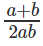B. (a + b) * 2ab

C.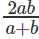D.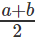Correct Answer is Option (A).

Given, log5x = a
log20x = b
logx5 = 1/a

logx20 = 1/b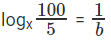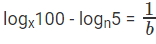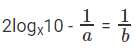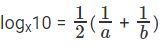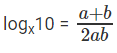The question is "What is logx10?"

##### Hence, the answer is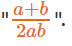Question 7: log3x + logx3 = 17/4. Find x.

A. 34

B. 31/8

C. 31/4

D. 31/3

Correct Answer is Option (C).

log3x + logx3 = 17/4

Let y = log3x
We know that logx3 =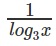Hence logx3 = 1/y

Thus the equation can be written as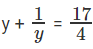4y2 + 4 = 17y
4y2 + 4 - 17y = 0
Solving the above equation we get y = 4 or 1/4

If y = 4
log3x = 4
Then x = 34
If y = 1/4

log3x = 1/4

Then x = 31/4
The question is "Find x."

##### Hence, the answer is "34".

Question 8: logxy + logyx2 = 3. Find logxy3.

A. 4

B. 3

C. 31/2

D. 31/16

Correct Answer is Option (B).

logxy + logyx2 = 3
Let a = logxy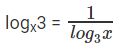logyx2 = 2logyx
We know that logyx =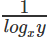Hence form above logyx = 1/a

Now rewritting the equation logxy + logyx2 = 3

Using a we get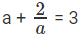i.e., a2 - 3a + 2 = 0
Solving we get a = 2 or 1
If a = 2, Then logxy = 2 and logyx3 = 3
logxy = 3 * 2 = 6
Or
If a = 1, Then logxy = 1 and logyx3 = 3
logxy = 3 * 1 = 3

The question is "Find logxy3."

##### Hence, the answer is "3".

Question 9: log2 4 * log4 8 * log8 16 * ……………nth term = 49, what is the value of n?

A. 49

B. 48

C. 34

D. 24

Correct Answer is Option (B).

First, the nth term of L.H.S need to be defined by observing the pattern :-
It is log(2n) 2.2n
Given,
log2 4 * log4 8 * log8 16 * ……………log(2n) 2.2n = 49
Whenever solving a logarithm equation, generally one should approach towards making the base same.
Making the base 2:-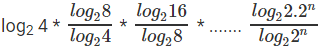log(2n) 2.2n = 49
log(2n) 2 + log(2n) 2n = 49
1 + n = 49
n = 48

The question is "what is the value of n?"

##### Hence, the answer is "48".

Question 10: If 33 + 6 + 9 + ……… 3x =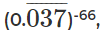what is the value of x?

A. 3

B. 6

C. 7

D. 11

Correct Answer is Option (D).

First of all, let us define the xth term.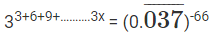Whenever you encounter a distinctive number such as one given in R.H.S of above equation, always try to find its significance in the context of question.

In this case L.H.S has 3a, so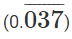must be some form of 3a.

With little hit and trial, you may find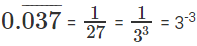33(1 + 2 + 3 + ...X) = 3 -3 * -66
33 * 3x(x+1)/2 = 33*66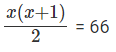x(x+1) = 132
Solving this equation for x > 0, we get x = 11.
You should directly be able to see that 132 = 11 * 12 => x= 11
And avoid wasting time solving the complete equation.

The question is "what is the value of x?"

##### Hence, the answer is "11".

Question 11: x, y, z are 3 integers in a geometric sequence such that y - x is a perfect cube. Given, log36x2 + log6√y + log216y1/2z = 6. Find the value of x + y + z.

A. 189

B. 190

C. 199

D. 201

Correct Answer is Option (A).

Let us begin with simplifying the equation:-
log62x2 + log6y1/2 + 3log63y1/2z = 6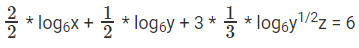log6x + log6y1/2y1/2z = 6
log6xyz = 6
xyz = 66

Given x,y,z is in G.P. Let x = a, y = ab, z = ab2
⇒ xyz = a3b3 = (ab)3
(ab)3 = (62)3
Possible values of (a,b) satisfying the equation :-
(1, 36), (2, 18), (3, 12), (4, 9), (9, 4), (12, 3), (18, 2), (36, 1)
Given y-x is a perfect cube
⇒ ab-a is perfect cube
⇒ a(b-1) is perfect cube
Only possible when (a, b) = (9, 4)
∴ x = 9 , y = 36 , z = 144
∴ x + y + z = 9 + 36 + 144 = 189

The question is "Find the value of x + y + z."

##### Hence, the answer is "189".

Question 1210log(3 - 10logy) = log2(9 - 2y), Solve for y.

A. 0

B. 3

C. 0 and 3

D. none of these

Correct Answer is Option (D).

Before beginning to simplify the equation, don’t forget that anything inside a log cannot be negative
10log(3-y) = log2(9 - 2y) (y > 0)…………………………………(1)
3 - y = log2(9 - 2y) (Therefore, 3 - y > 0 =) (y < 3)) ……………………… (2)
23-y = 9 - 2y
2y = t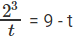⇒ 8 = 9t –t2
⇒ t2 - 9t + 8 = 0
⇒ t2 - t - 8t - 8 = 0
⇒ t(t - 1) - 8(t - 1) = 0
⇒ t = 1, 8
Therefore, 2y = 1 and 2y = 8
⇒ y = 0 and y = 3
However, from inequalities (1) and (2), y cannot take either of these value.

The question is "Solve for y."

##### Hence, the answer is "none of these".

Question 13: 46+12+18+24+…+6x = (0.0625)-84, what is the value of x?

A. 7

B. 6

C. 9

D. 12

Correct Answer is Option (A).

Take right side expression,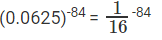= (4-2)-84
= 4168
Take left side expression
46+12+18+24+…+6x = 46(1+2+3+4+x)
= 46 * 4(1+2+3+4+…+x)
= 46 * 4x(x+1)/2 (using the formula for sum of natural numbers from 1 to x)
Equating left and right side expresssions, we get 46 * 4x(x+1)/2 = 4168
Or 46 * 4x(x+1)/2 = 46*28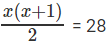or x (x + 1) = 56
Solving for x we get, x = 7

The question is "what is the value of x?"

##### Hence, the answer is "7".
The document Important Formulas: Logarithms Notes | Study Quantitative Aptitude (Quant) - CAT is a part of the CAT Course Quantitative Aptitude (Quant).
All you need of CAT at this link: CAT

## Quantitative Aptitude (Quant)

163 videos|164 docs|131 tests
 Use Code STAYHOME200 and get INR 200 additional OFF

## Quantitative Aptitude (Quant)

163 videos|164 docs|131 tests

### How to Prepare for CAT

Read our guide to prepare for CAT which is created by Toppers & the best Teachers

Track your progress, build streaks, highlight & save important lessons and more!

,

,

,

,

,

,

,

,

,

,

,

,

,

,

,

,

,

,

,

,

,

;